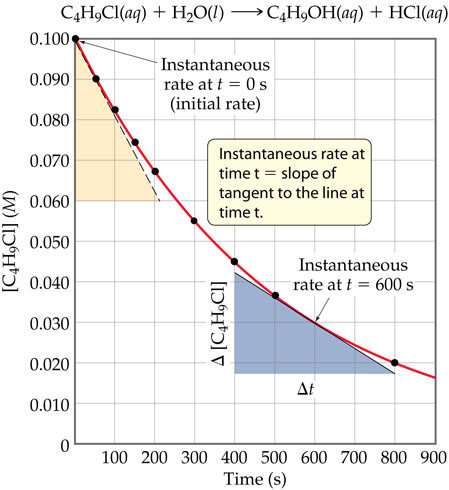# Problem: Concentration of butyl chloride (C4H9Cl) as a function of time.In the figure on the left, order the following three rates from fastest to slowest: (i) The average rate of the reaction between 0 s and 600 s, (ii) the instantaneous rate at t = 0 s, and (iii) the instantaneous rate at t = 600 s. You should not have to do any calculations.

🤓 Based on our data, we think this question is relevant for Professor Daly's class at UD.

###### FREE Expert Solution

We are asked to order three rates from fastest to slowest:

(i) The average rate of the reaction between 0 s and 600 s, (ii) the instantaneous rate at t = 0 s, and (iii) the instantaneous rate at t = 600 s.

In the graph, it is shown that the concentration of C4H9Cl was measured at various times.###### Problem DetailsConcentration of butyl chloride (C4H9Cl) as a function of time.

In the figure on the left, order the following three rates from fastest to slowest:

(i) The average rate of the reaction between 0 s and 600 s

(ii) the instantaneous rate at t = 0 s, and

(iii) the instantaneous rate at t = 600 s

You should not have to do any calculations.